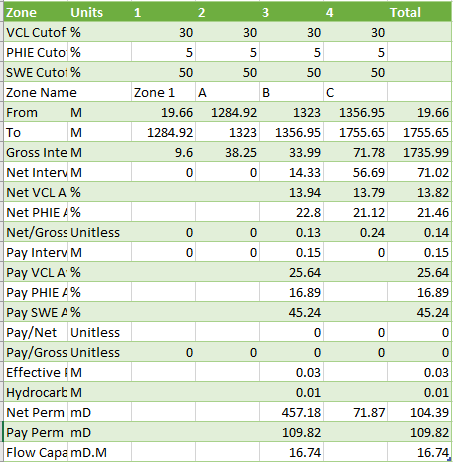top of page
Search
•Nicholas Harvey

# LogScope Petrophysical Capabilities

Harvey Rock Physics has released the latest version of the LogScope software with the following capabilities:

• Project window complimented by map window

• Cross section capability added - can plot up to 533 wells

• Tadpole for formation dip and azimuth added to basic display plots

• Basic log analysis now has Carbonate origin in addition to clastic for UMTA-RHOMA cross plot.

• Insertion of special minerals

• Nphi matrix reference can be set by zone (i.e Limestone, Sandstone and Dolomite)

• DPHI can be used to back calculate the density

• Inclusion of deterministic mineral model (DMM), silty shaley sand (SSS) and dual or two water model (TWA):

• These models can be selected and used by zone

• Basic model is similar to DMM but when the PEF is bad or not available it uses a simple matrix lithology model.

• Variable M and N entry

• Geomechanical model computing moduli and other additional parameters

• Shale gas model computing TOC and Shale Gas volume using a variety of methodologies

• Coal Bed Methane (CBM) computation

• Hydrocarbon Volume Report

• Undo/Redo

• LAS 3 import and export

The module adds to the BASIC log analysis module a Deterministic Mineral Model, a Silty Shaley Sand Model and a Dual water model. Any combination of modules can be used in a well by specifying the model required in each zone.

The Density Neutron, Sonic Neutron, Density Sonic and M factor N Factor cross plots are all interlinked so when the parameters move on one cross plot they move on the others. The figure below illustrates the interconnected nature of the parameters using Density, Neutron and Sonic cross plots typically used to identify porosity.The deterministic mineral model or DMM model uses the PEF and Density log to make the determination of lithology type as per the following cross plot. This model still computes a PHIE and PHIT as well as associated saturation curves. It uses a variable matrix density.The Silty Shale Sand model uses the Neutron-Density cross plot to allow the user to specify a silt point. An example of the cross plot is shown below. There is added points for sand (red) and silt (blue)The results of the SSS model is shown for zone B in the figure below. A and C are left using the DMM for comparison.The two water model or dual water model (TWA) allows the user to specify a different resistivity for water in the clays and it uses this to compute the results. The example below shows the calculation with some hydrocarbon added to the clay interval in zone B just to illustrate.The inclusion of Rock Mechanics module allows the computation of rock moduli. There is also a module that computes TOC, shale gas etc as shown in the example plot below.The tracks from left to right are:

1. Depth

2. Gamma ray

4. resistivity

5. Density, Neutron, Sonic and PEF

6. Volumetric build out of lithology from logs

7. Saturation

8. Porosity

9. TOC/Kerogen

10. Recomputed compressional sonic and shear sonic

11. Rock Moduli

12. UCS with fracture modulus as color infill

13. Shale gas.The HVR produces the following results in a comma delimited file. The user has control over Clay, Porosity and Saturation cutoffs and can output by clean formation or zone.To trial these and any other features one can download Logscope from here